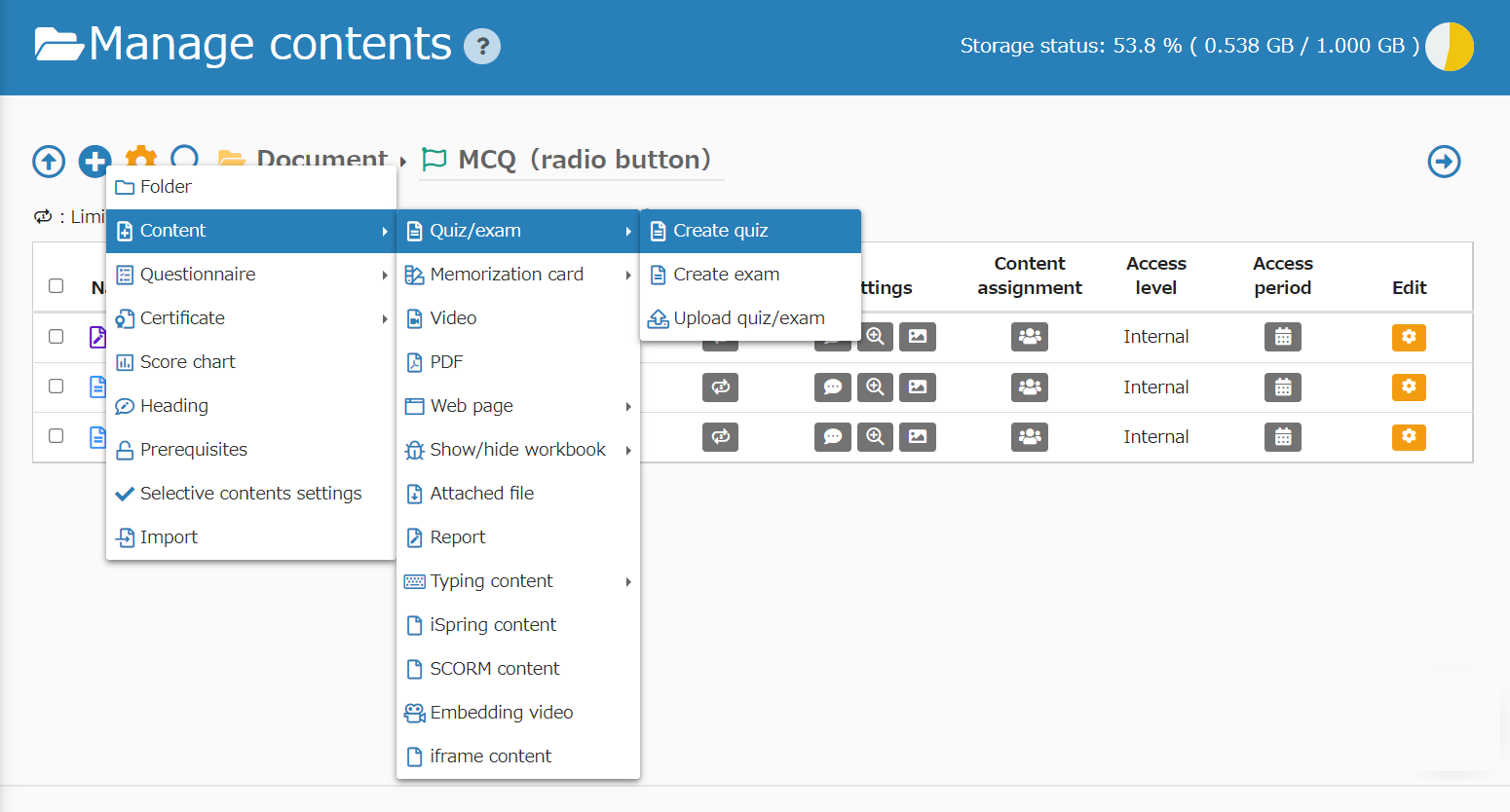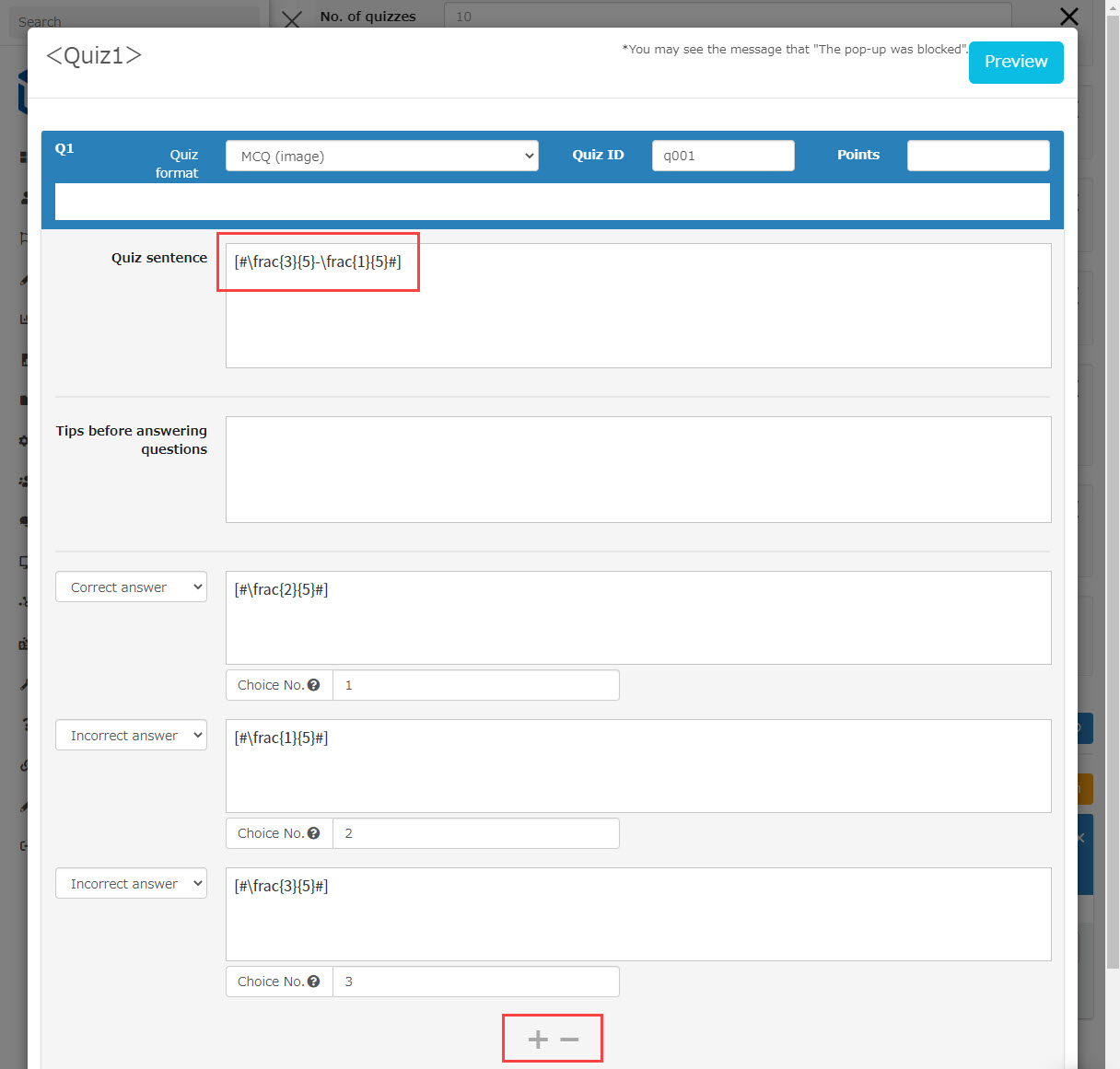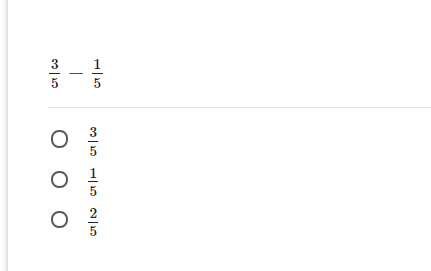# Formula

### Formula

QuizGenerator can create questions with mathematical expressions. If you write a formula between [# and #] in LaTeX format, it will be converted to an image and displayed in the question.

You can also see an example of inputting a mathematical expression in LaTeX format at the following site.

### How to Create a Mathematical Problem

• text
• Excel
• Quiz/exam creation form

#passing_score:50
#time_limit:300
#messages_intro: problem start!
What is the sum of the interior angles of a pentagon?
sa:
[#540^\circ#]
[#360^\circ#]
[#450^\circ#]

[#(3\times3)-(6\div2)#]
sa:
6
3
2

[#3^{3}-2^{3}#]
sa:
19
13
5

[#(6\times7)-(25\div5)#]
sa:
37
27
17

What is the sum of the interior angles of a parallelogram?
sa:
[#105^\circ#]
[#205^\circ#]
[#115^\circ#]

[#(8\times8)-(72\div9)#]
sa:
56
46
66

[#\frac{3}{5}-\frac{1}{5}#]
sa:
[#\frac{2}{5}#]
[#\frac{1}{5}#]
[#\frac{3}{5}#]

 A b C d e 1 #format:table sa: 2 What is the sum of the interior angles of a pentagon? sa: [#540^\circ#] [#360^\circ#] [#450^\circ#] 3 [#(3\times3)-(6\div2)#] sa: 6 3 2 4 [#3^{3}-2^{3}#] sa: 19 13 5 5 [#-8+\{3-(-2^{3})}\times4-(4^{2}+5)\div(-3)#] sa: 43 32 37 6 Find the square root of the following number. sa: [#↩pm484#] [1am3pm221stp3t] [1tps3t pm181tps3t]
You can also create questions that use mathematical formulas.

As shown in the red frame of the question, if you write a mathematical expression in LaTeX style between [# and #], it will be converted into an image and displayed in the question.

Click on the menu button (+ button) in Content Management → Materials → Quizzes and Tests → "Create quiz" or "Create exam".Click on "Edit Question" and select the question type.

Fill in the question text and choices in LaTeX format.
The + button in the red frame allows you to add choices, and the - button allows you to delete choices.The following image shows the completed equation problem.